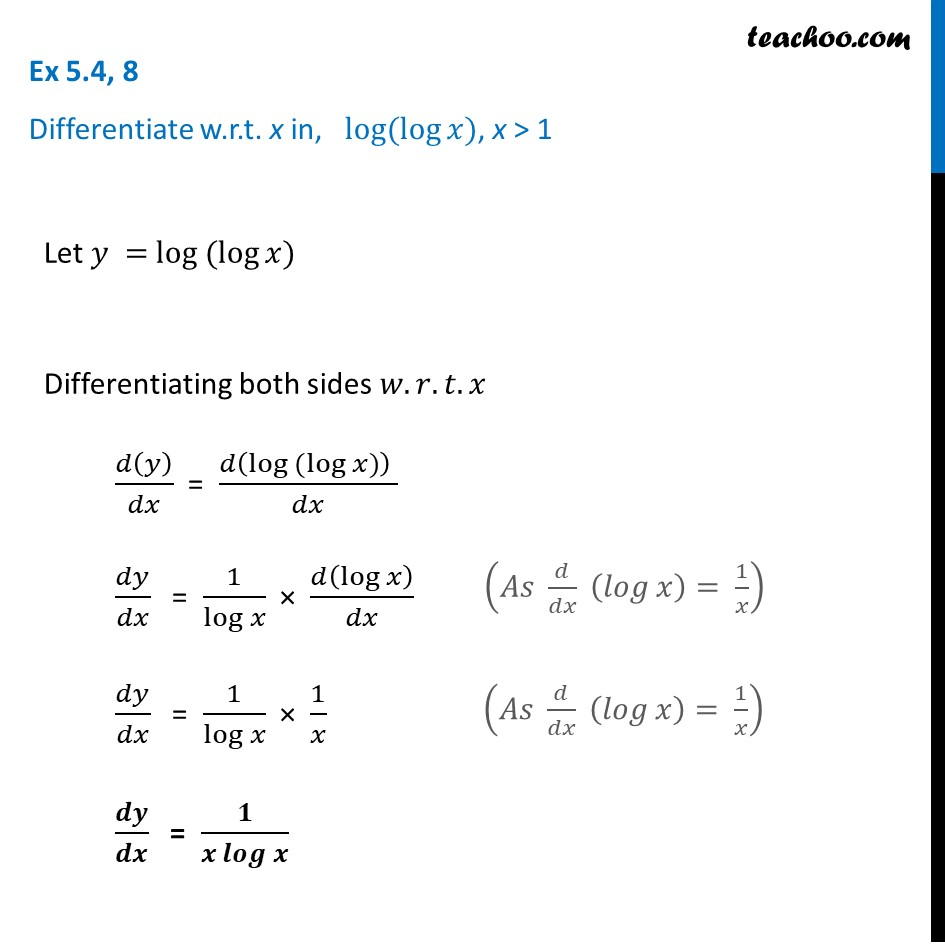Finding derivative of Exponential & logarithm functions

Chapter 5 Class 12 Continuity and Differentiability
Concept wiseLearn in your speed, with individual attention - Teachoo Maths 1-on-1 Class

### Transcript

Ex 5.4, 8 Differentiate w.r.t. x in, log⁡(log⁡𝑥), x > 1Let 𝑦 = log ⁡(log⁡𝑥) Differentiating both sides 𝑤.𝑟.𝑡.𝑥 𝑑(𝑦)/𝑑𝑥 = (𝑑(log ⁡(log⁡𝑥)) )/𝑑𝑥 𝑑𝑦/𝑑𝑥 = 1/log⁡𝑥 × 𝑑(log⁡𝑥)/𝑑𝑥 𝑑𝑦/𝑑𝑥 = 1/log⁡𝑥 × 1/𝑥 𝒅𝒚/𝒅𝒙 = 𝟏/(𝒙 𝒍𝒐𝒈⁡𝒙 ) (𝐴𝑠 𝑑/𝑑𝑥 (𝑙𝑜𝑔⁡𝑥 )= 1/𝑥) (𝐴𝑠 𝑑/𝑑𝑥 (𝑙𝑜𝑔⁡𝑥 )= 1/𝑥)